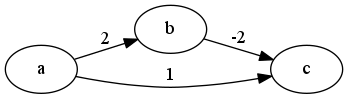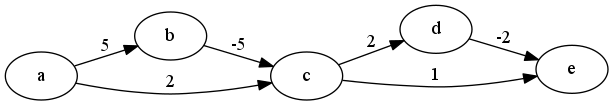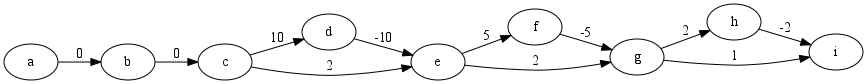# Internet Problem Solving Contest

## Solution to Problem D – Dijkstra's Nightmare

Below is the smallest example of a graph that will force Dijkstra’s algorithm to evaluate the same vertex (in this case, vertex c) twice. The algorithm will process the vertices in the following order: a at distance 0, c at distance 1, b at distance 2, and then c again at distance 0.We can now concatenate two such blocks, as follows:This will force the algorithm to recompute the last vertex 4 times: at distance 3, then 2, then 1, and finally 0. The above pattern can be easily generalized to an arbitrary length; the number of times the last vertex is recomputed will always be 2t, where t is the number of triangles we used. A long enough pattern like this is sufficient to solve the easy subproblem.

(Note that the edge lengths used in the above construction also grow exponentially in the number of triangles. This is actually necessary in any valid solution to our problem – if all the edge lengths are, say, polynomial in n, all the distances computed during the algorithm will also be polynomial in n, and therefore the total number of updates will also be polynomial in n.)

Tweaking the construction to solve the hard subproblem is not that hard. Here’s one possibility: Note the leftmost horizontal edge (edge ac in our picture above). By decreasing its length we can very smoothly decrease the number of vertices processed by the algorithm. In order to construct a graph with a specific number of processed vertices, we can now:

1. Find the smallest number of triangles that produces at least the necessary number of processed vertices.
2. Decrease the length of the first horizontal edge to make the number of processed vertices either exact or slightly smaller than what we need.
3. If necessary, prepend a chain of vertices that will only get processed once, at the beginning.

Below is an example for p = 20 processed vertices. Note that the length of the edge ce has been decreased from 4 to 2.